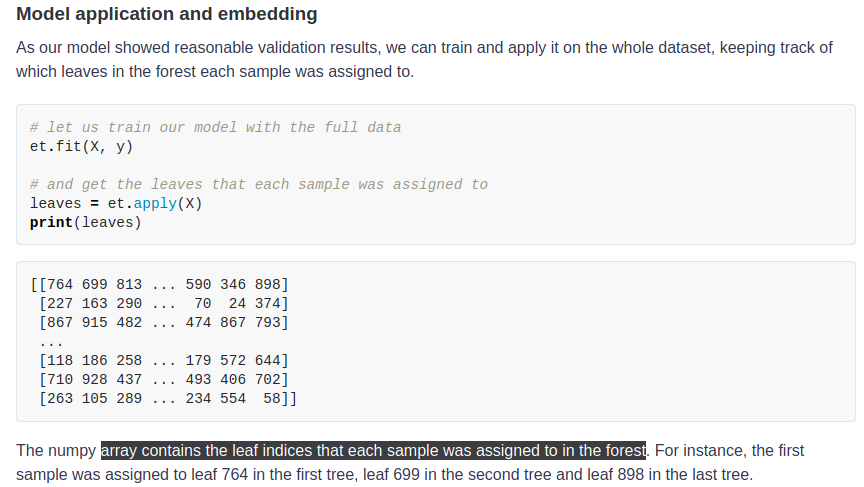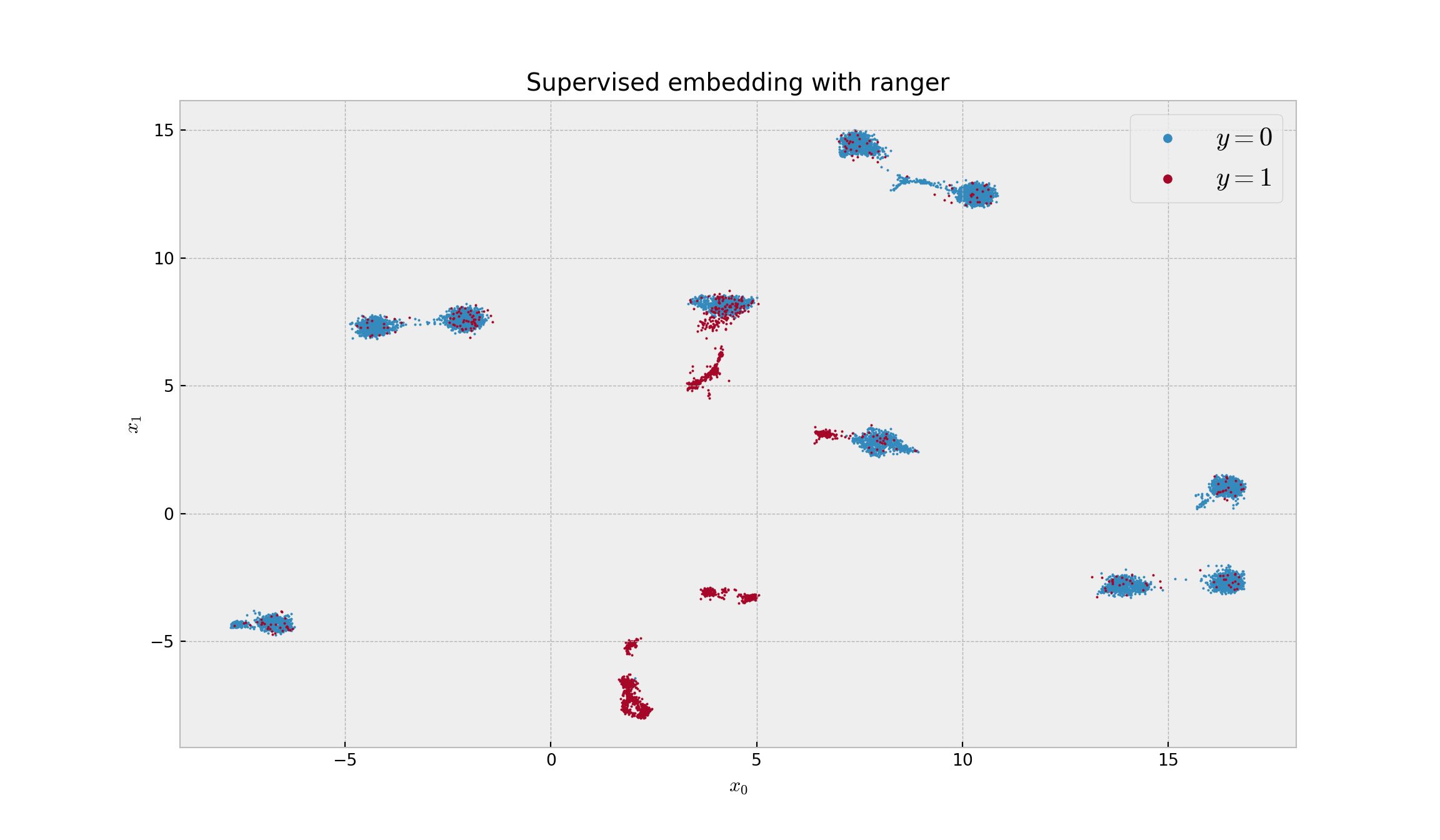# Project Overview

I’ve transcompiled code from `Python` (code shown in red) to `R`(code shown in blue) in order to do supervised dimensionality reduction with Random Forests (RF) and UMAP following this blog post.

The goal was to get an array that contains the leaf indices that each sample was assigned to in the forest in order to feed this information into {`uwot`} (for `UMAP` implementation in `R`).

I tried out the following `R` packages, {randomForest}, {ranger}, and {extraTrees}. {`ranger`} was expected to require the lowest compute-time (critical for my project) while {`extraTrees`}, in some-cases, can outperform RF implementations (in terms of AUC). _Note: For this project, we are providing a `Java` facing UI to clinicians who are Subject Matter Experts who have background knowledge in the underlying biology of an analysis but lack the computational expertise for such analysis. Also note, we are limited to `1 core` on the Java server we provide for analysis; so, necessarily we will benchmark tools in `serial` below.

In `Python`, `scikit-learn` the `ExtraTreesClassifier` returns a `numpy` array which contains the leaf indices that each sample was assigned to in the forest like so:To make it as comparable as-possible between `R`/`Python` we will do the brunt of the work using `Python` using {`reticulate`} following instructions from the blog (however, here, we are decreasing the size of simulated data) comparing only the various RF implementations.

# Setting up the `Python` environment

``````reticulate::conda_create('r-reticulate', python_version = 3.6)
PYTHON_DEPENDENCIES = c('pandas',
'numpy',
'numba',
'scipy',
'matplotlib',
'scikit-learn',
'umap-learn',
'tqdm',
'pynndescent',
'fastcluster'
)
reticulate::conda_install('r-reticulate', packages = PYTHON_DEPENDENCIES, ignore_installed=TRUE)``````

## Python Implementation

``````import numpy as np
import pandas as pd
import scipy as sp
import matplotlib.pyplot as plt
from sklearn.ensemble import RandomForestClassifier, ExtraTreesClassifier
from sklearn.preprocessing import OneHotEncoder, StandardScaler
from sklearn.model_selection import cross_val_predict, StratifiedKFold
from sklearn.metrics import roc_auc_score
from sklearn.datasets import make_classification
from tqdm import tqdm
from umap import UMAP  ## if this doesn't work try: import umap.umap_ as UMAP
from pynndescent import NNDescent
from fastcluster import single
from scipy.cluster.hierarchy import cut_tree, fcluster, dendrogram
from scipy.spatial.distance import squareform
from sklearn.tree import DecisionTreeClassifier, ExtraTreeClassifier
from sklearn.model_selection import train_test_split

# turning off automatic plot showing, and setting style
plt.style.use('bmh')

# let us generate some data with 10 clusters per class
X, y = make_classification(n_samples=10000, n_features=500, n_informative=5,
n_redundant=0, n_clusters_per_class=10, weights=[0.80],
flip_y=0.05, class_sep=3.5, random_state=42)

# normalizing to eliminate scaling differences
X = pd.DataFrame(StandardScaler().fit_transform(X))

# Split dataset into training set and test set
X_train, X_test, y_train, y_test = train_test_split(X, y, test_size=0.3, random_state=42) # 70% training and 30% test``````

Let’s use `Python`’s `ExtraTreesClassifier` to get the information from leaf indices and plot.

In the blog post the author used `StratifiedKFold` & `cross_val_predict`. Here I use `train_test_split` instead to create a train/test split. This way I can use the same train/split for `Python` & `R` and ensure the Area under the ROC curve measurement is comparable.

``````# model instance
et = ExtraTreesClassifier(n_estimators=100, min_samples_leaf=500, max_features=0.80, bootstrap=True, class_weight='balanced', n_jobs=-1, random_state=42)

# Train ExtraTreesClassifer
et.fit(X_train, y_train)

# Print the AUC``````
``````## ExtraTreesClassifier(bootstrap=True, class_weight='balanced', max_features=0.8,
##                      min_samples_leaf=500, n_jobs=-1, random_state=42)``````
``print('Area under the ROC Curve:', roc_auc_score(y_test, et.predict_proba(X_test)[:,1]))``
``## Area under the ROC Curve: 0.850375887423935``

The models performance is 0.8504 AUC. Now we move on to embedding.

``````# let us train our model with the full data
et.fit(X, y)

# and get the leaves that each sample was assigned to``````
``````## ExtraTreesClassifier(bootstrap=True, class_weight='balanced', max_features=0.8,
##                      min_samples_leaf=500, n_jobs=-1, random_state=42)``````
``leaves = et.apply(X)``

What are dimensions of the `numpy` array?

``leaves.shape``
``## (10000, 100)``
``````# calculating the embedding with hamming distance
sup_embed_et = UMAP(metric='hamming').fit_transform(leaves)

# plotting the embedding``````
``````## /home/mtg/miniconda3/envs/r-reticulate/lib/python3.6/site-packages/umap/umap_.py:1762: UserWarning: gradient function is not yet implemented for hamming distance metric; inverse_transform will be unavailable
##   "inverse_transform will be unavailable".format(self.metric)``````
``plt.figure(figsize=(12,7), dpi=150)``
``plt.scatter(sup_embed_et[y == 0,0], sup_embed_et[y == 0,1], s=1, c='C0', cmap='viridis', label='\$y=0\$')``
``## <matplotlib.collections.PathCollection object at 0x7f1b12fefc50>``
``plt.scatter(sup_embed_et[y == 1,0], sup_embed_et[y == 1,1], s=1, c='C1', cmap='viridis', label='\$y=1\$')``
``## <matplotlib.collections.PathCollection object at 0x7f1b12d247f0>``
``plt.title('Supervised embedding with ExtraTrees')``
``## Text(0.5, 1.0, 'Supervised embedding with ExtraTrees')``
``plt.xlabel('\$x_0\$'); plt.ylabel('\$x_1\$')``
``````## Text(0.5, 0, '\$x_0\$')
## Text(0, 0.5, '\$x_1\$')``````
``````plt.legend(fontsize=16, markerscale=5);
plt.show()````````````# taking a sample of the dataframe
embed_sample = pd.DataFrame(sup_embed_et).sample(5000, random_state=42)

# running fastcluster hierarchical clustering on the improved embedding
H = single(embed_sample)

# getting the clusters
clusters = cut_tree(H, height=0.35)
print('Number of clusters:', len(np.unique(clusters)))``````
``## Number of clusters: 19``

This shows 19 clusters (the simulated number was 20).

``````# creating a dataframe for the clustering sample
clust_sample_df = pd.DataFrame({'cluster': clusters.reshape(-1), 'cl_sample':range(len(clusters))})

# creating an index with the sample used for clustering
index = NNDescent(embed_sample, n_neighbors=10)

# querying for all the data
nn = index.query(sup_embed_et, k=1)

# creating a dataframe with nearest neighbors for all samples
to_cluster_df = pd.DataFrame({'sample':range(sup_embed_et.shape), 'cl_sample': nn.reshape(-1)})

# merging to assign cluster to all other samples, and tidying it
final_cluster_df = to_cluster_df.merge(clust_sample_df, on='cl_sample')
final_cluster_df = final_cluster_df.set_index('sample').sort_index()

# plotting the embedding
plt.figure(figsize=(12,7), dpi=150)``````
``plt.scatter(sup_embed_et[:,0], sup_embed_et[:,1], s=1, c=final_cluster_df['cluster'], cmap='plasma')``
``## <matplotlib.collections.PathCollection object at 0x7f1b40c6ee48>``
``plt.title('Hierarchical clustering and extraTrees')``
``## Text(0.5, 1.0, 'Hierarchical clustering and extraTrees')``
``plt.xlabel('\$x_0\$'); plt.ylabel('\$x_1\$')``
``````## Text(0.5, 0, '\$x_0\$')
## Text(0, 0.5, '\$x_1\$')``````
``plt.show()``